Practice the AP 10th Class Physical Science Bits with Answers Chapter 9 Electric Current on a regular basis so that you can attempt exams with utmost confidence.

## AP State Syllabus 10th Class Physical Science Bits 9th Lesson Electric Current with Answers

CONCEPT – 1 : Electric Current, Potential Difference & emf

Question 1.
What type of charge flows through an electric wire when it is connected in an electric circuit ?
A) positive
B) negative
C) either
D) neither
B) negative

Question 2.
Which of the following phenomena is an evidence for the motion of charge in daily life situations ?
A) Formation of rainbow in the sky
B) Formation of mirages
C) Lightning between two clouds
D) None
C) Lightning between two cloudsQuestion 3.
Which of the following situations makes the bulb glows ?
A) When we connect the ends of copper wire to the terminals of the battery through the bulb and switch to make a complete circuit and switch on the circuit
B) When we connect the copper wire, bulb and switch to make a complete circuit and switch on the circuit.
C) When we connect the nylon wire to the terminals of the battery through the bulb and switch to make complete circuit and switch on the circuit.
D) none.
A) When we connect the ends of copper wire to the terminals of the battery through the bulb and switch to make a complete circuit and switch on the circuit

Question 4.
In an electric cell
A) chemical energy converts into electrical energy
B) mechanical energy converts into electrical energy
C) electrical energy converts into mechanical energy
D) electrical energy converts into chemical energy
A) chemical energy converts into electrical energy

Question 5.
Statement X : The material which transfers energy from battery to the bulb is called conductor
Statement Y : The material which cannot transfer energy from battery to the bulb is called insulator.
A) Both statements are true
B) Both statements are false
C) X is true, Y is false
D) X is false, Y is true
A) Both statements are true

Question 6.
Name the scientist who proposed that metal conductors contain a large number of free electrons.
A) Drude
B) Loreritz
C) Both Drude & Lorentz
D) Ohm
C) Both Drude & Lorentz

Question 7.
The arrangement of the positive ions in a metal conductor is called ……………………
A) free electrons
B) bounded electrons
C) lattice
D) none
C) lattice

Question 8.
The net charge flowing through a crosssection of a conductor in unit time is …………………. when the conductor is in open circuit.
A) electric current
B) one ampere
C) onecoloumb
D) zero
D) zero

Question 9.
Which of the following statement is false?
A) When the electrons are in ordered motion, there will be a net charge crossing through any cross-section of the conductor.
B) When the electrons are in random motion, there will be no net charge crossing through any cross section of the conductor.
C) The positive ions of the metal conductor are fixed in their location.
D) The positive ions of the metal conductor are in motion.
D) The positive ions of the metal conductor are in motion.Question 10.
Which of the following statements is not correct ?
A) Electric current is ordered motion of charges.
B) The amount of charge crossing any cross section of the conductor in one second is called electric current.
C) The amount of one coloumb charge crossing any cross section of the conductor in one second is called one ampere
D) Electric current is random motion of free electrons in a conductor.
D) Electric current is random motion of free electrons in a conductor.

Question 11.
The equation for an electric current is ……………….
A) I = $$\frac{\mathrm{Q}}{\mathrm{t}}$$
B) I = Q × t
C) I = $$\frac{t}{Q}$$
D) I = $$\frac{\mathrm{Q}}{\mathrm{t}^{2}}$$
A) I = $$\frac{\mathrm{Q}}{\mathrm{t}}$$

Question 12.
The S.I unit of electric current is …………………
A) volt
B) ampere
C) oersted
D) ohm
B) ampere

Question 13.
1 coloumb /1 second = ………………….
A) one volt
B) one ohm
C) one ampere
D) one watt
C) one ampere

Question 14.
Statement X : The free electrons in the conductor are accelerated by the electric field and move in a direction opposite to the direction of the electric field.
Statement Y : The free electrons in the conductor are accelerated by the electric field and move in a direction parallel to the direction of the electric field.
A) Both statements are true
B) Both statements are false
C) X is true, Y is false
D) X is false, Y is true
C) X is true, Y is false

Question 15.
Electrons in the conductor move with a constant average speed. This speed is called ………………….
A) drift speed
B) drift velocity
C) either A or B
D) neither A nor B
C) either A or B

Question 16.
The magnitude of electric charge is ……………………….
A) 1.602 × 1019 C
B) 1.602 × 10-19C
C) 8.5 × 1028C
D) 7 × 10-5C
B) 1.602 × 10-19CQuestion 17.
The S.I unit of an electric charge is ………………..
A) coloumb
B) ampere
C) volt
D) second
A) coloumb

Question 18.
Statement X : The conventional direction of electric current is from positive terminal of a cell to the negative terminal through the outer circuit.
Statement Y : The actual direction of electric current is from negative terminal of a cell to the positive terminal through the outer circuit.
A) Both statements are true
B) Both statements are false
C) X is true, Y is false
D) X is false, Y is true
A) Both statements are true

Question 19.
Match the following.

 1) Electric current (I) a) It 2) Average drift speed (Vd) b) I/nqA 3) Electric charge (Q) c) nqAvd

A) 1 → a, 2 → b, 3 → c
B) 1 → b, 2 → c, 3 → a
C) 1 → c, 2 → b, 3 → a
D) 1 → c, 2 → a, 3 → b
C) 1 → c, 2 → b, 3 → a

Question 20.
Calculate the drift speed of an electron in a copper wire carrying a current of 1 A and cross sectional area A = 10-6m2 [n = 8.5 × 1028 m-3]
A) 0.07 cm/s
B) 0.07 m/s
C) 7 x 10-5m/s
D) Both B and C
D) Both B and C

Question 21.
Electric current can be measured by …………………
A) a voltmeter
B) an ammeter
C) a cell
D) a resistor
B) an ammeter

Question 22.
An ammeter is always connected in ……………… to the circuit
A) series
B) parallel
C) both series (or) parallel
D) none
A) series

Question 23.
The amount of work done in moving a unit charge from one point to the other point in an electric circuit is called …………………..
A) potential difference
B) voltage
C) both A and B
D) wattage
C) both A and B

Question 24.
The equation for potential difference is ………………….
A) $$\frac{\mathrm{W}}{\mathrm{q}}$$
B) V = Wq
C) V = W ÷ q
D) V = W + q
A) $$\frac{\mathrm{W}}{\mathrm{q}}$$Question 25.
The S.I unit of potential difference is ………………….
A) ampere
B) ohm
C) coloumb
D) volt
D) volt

Question 26.
1 Joule /1 Coloumb = ……………………….
A) 1 ampere
B) 1 ohm
C) one volt
D) one coloumb
C) one volt

Question 27.
Statement X : In case of metal conductors there will be only motion of negative charges.
Statement Y : In case of liquid conductors there will be only motion of positive charges.
A) Both statements are true
B) Both statements are false
C) X is true, Y is false
D) X is false, Y is true
C) X is true, Y is false

Question 28.
Electrons move in this direction ………………….
A) higher potential to lower potential
B) lower potential to higher potential
C) higher temperature to lower temperature
D) lower temperature to higher temperature
B) lower potential to higher potential

Question 29.
The potential difference between two points separated by a distance l in a conductor wire by the electric force on a free charge ‘q’ is …………………….
A) V= $$\frac{\mathrm{F}}{l \mathrm{q}}$$
B) V = $$=\frac{l q}{F}$$
C) V = $$\frac{\text { F.l }}{\mathrm{q}}$$
D) none
C) V = $$\frac{\text { F.l }}{\mathrm{q}}$$

Question 30.
The potential difference is measured by an instrument called ……………………
A) voltmeter
B) ammeter
C) ohm-meter
D) galvanometer
A) voltmeter

Question 31.
The voltmeter is always connected in ………………….. across the two points where the potential difference to be measured.
A) series
B) parallel
C) either
D) neither
B) parallelQuestion 32.
Statement X : The voltmeter is always connected in parallel and it has a high resistance so that it takes negligible current from the circuit
Statement Y : The ammeter is always connected in series and it has a low resistance so that it may not change the current flowing in the circuit.
A) Both statements are true
B) Both statements are false
C) X is true, Y is false
D) X is false, Y is true
A) Both statements are true

Question 33.
The e.m.f of a” battery is measured by an instrument called
A) ammeter
B) ohm-meter
C) voltmeter
D) galvanometer
C) voltmeter

Question 34.
If a charge q is moving against an electric force Fe through a distance “d” between the terminals of a battery then the em/is ……………………..
A) Fe.dq
B) Fe/dq
C)Fed/q
D)Feq/d
C)Fed/q

Question 35.
The quantity which has SI unit as coloumb is ……………………..
A) Electric current
B) Electric capacitance
C) Electric charge
D) Electric potential
C) Electric charge

Question 36.
A charge is moved from a point A to point B. The work done to move an unit charge during this process is called ……………………
A) potential at A
B) potential at B
C) potential difference between A and B
D) current from A to B
C) potential difference between A and B

CONCEPT – II : Ohm’s law, Factors affecting the resistance of a material and series, parallel connection of resistors :

Question 37.
The potential difference between the ends of a conductor is directly proportional to the electric current passing through it at constant temperature. This law is known as …………….
A) Ohm’s law
B) Joule’s law
C) Power’s law
A) Ohm’s lawQuestion 38.
Which of the following is an equation for Ohm’s law ?
A) V = IR
B) I = $$\frac{\mathrm{V}}{\mathrm{R}}$$
C) R = $$\frac{\mathrm{V}}{\mathrm{I}}$$
D) All
D) All

Question 39.
The S.I unit of resistance is ……………………
A) volt
B) ohm
C) ampere
D) coloumb
B) ohm

Question 40.
1 volt/1 ampere = ………………….
A) 1 coloumb
B) 1 joule
C) 1 watt
D) 1 ohm
D) 1 ohm

Question 41.
The ratio of potential difference to current is ………………….
A) voltage
B) specific resistance
C) current
D) resistance
D) resistance

Question 42.
Statement X: The materials which obey Ohm’s law are called ohmic materials.
Statement Y : The materials which do not obey ohm’s law are called non-ohmic conductors.
A) Both statements are true
B) Both statements are false
C) X is true, Y is false
D) X is false, Y is true
A) Both statements are true

Question 43.
Ohm’s law is valid for …………………. conductors provided the temperature and other physical conditions remain constant.
A) gaseous
B) semiconductors
C) metal
D) none
C) metal

Question 44.
Which of the following is a non-ohmic conductor?
A) germanium
B) silicon
C) LED
D) All
D) AllQuestion 45.
Electrical resistance of a conductor was first determined by
A) Coloumb
C) George simon Ohm
D) Ampere
C) George simon Ohm

Question 46.
The V-I graph for a metal conductor will be …………………. when provided the temperature
and other physical conditions remain constant
A) non-linear
B) linear
C) parabola
D) curved graph
B) linear

Question 47.
Which of the following V-I graph represents ohmic conductor ……………………….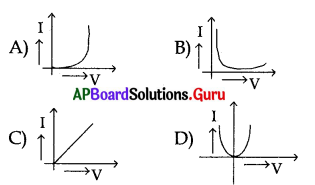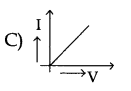Question 48.
The substance having infinitely high electrical resistance is called ………………..
A) conductor
B) resistor
C) super conductor
D) insulator
D) insulator

Question 49.
Keeping the potential difference constant, the resistance of a circuit is doubled. The current will become
A) double
B) half
C) one-fourth
D) four-times
B) half

Question 50.
Keeping the p.d constant, the resistance of a circuit is halved. The current will become
A) one-fourth
B) four times
C) half
D) double
D) double

Question 51.
The p.d across a 3 Ω resistor is 6 V. The current flowing in the resistor will be ……………………..
A) $$\frac{1}{2}$$A
B) 3A
C) 2A
D) 6A
C) 2AQuestion 52.
When a 4 Ω resistor is connected across the terminal of a 12 V battery, the number of coloumbs passing through the resistor per second is …………………..
A) 0.3
B) 8
C) 3
D) 12
C) 3

Question 53.
Find the resistance of a wire from the V-I graph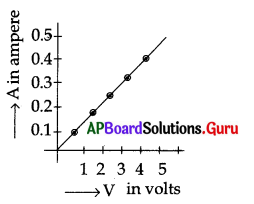A) 10 Ω
B) 20 Ω
C) 30 Ω
D) 1 Ω
A) 10 Ω

Question 54.
Find the current passing through the wire when p.d across a wire is 5 V from the V-I graph
A) 0.3 A
B) 0.1 A
C) 0.5 A
D) 5 A
C) 0.5 A

Question 55.
Find the potential difference across a wire when the current passing through a wire is 0.8
A) 5 V
B) 8 V
C) 6 V
D) not defined
B) 8 V

Question 56.
The above V-I graph represents …………………. conductor.
A) semi
B) gaseous
C) metallic
D) not defined
C) metallic

Question 57.
Statement X : The obstruction to the motion of free electrons in a conductor is called resistance of the conductor.
Statement Y : The material which offers resistance to the motion of electrons is called resistor.
A) Both statements are true
B) Both statements are false
C) X is true, Y is false
D) X is false, Y is true
A) Both statements are true

Question 58.
The V-I graph of two conductors of resistances and R2 are shown by A and B in the figure. Which is having greater resistance.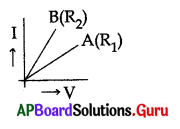A) R1
B, R2
C) R1 = R2
D) not defined
A) R1Question 59.
Match the following.

 Current in ampere Effect 1) 0.001 a) It does not affect the functioning of organs 2) 0.00024 b) Can be felt 3) 0.005 c) It can affect the functioning of heart 4) 0.07 d) It is painful

A) 1 → a, 2 → b, 3 → c, 4 → d
B) 1 → d, 2 → c, 3 → b,4 → a
C) 1 → b, 2 → a, 3 → d, 4 → c
D) 1 → b, 2 → a, 3 → c, 4 → d
C) 1 → b, 2 → a, 3 → d, 4 → c

Question 60.
A bird does not get an electric shock when it stands on a high voltage single wire because
A) the body of a bird is an electric insulator
B) the current that passes through the body of a bird does not affect the organs inside the body.
C) there is no potential difference between the legs of a bird
D) none
C) there is no potential difference between the legs of a bird

Question 61.
Statement X : The disturbance of the functioning of organs inside the human body is felt as electric shock Statement Y : The skin offers more resistance than the organs inside the human body.
A) Both statements are true
B) Both statements are false
C) X is true, Y is false
D) X is false, Y is true
A) Both statements are true

Question 62.
The electric shock felt by a human body depends on ………………..
A) potential difference
B) electric current
C) resistance
D) all
D) all

Question 63.
The electrical resistance of a conductor depends on …………………….
a) temperature of the conductor
b) nature of the material of the conductor
c) length of the conductor
d) area of cross-section of the conductor
A) onlyb
B) a, b and c
C) b, c and d
D) a, b, c and d
D) a, b, c and d

Question 64.
The value of a resistance of a bulb …………………. when the temperature of a filament in a bulb increases for providing constant potential difference
A) increases
B) decreases
C) does not depend on temperature
D) none
A) increases

Question 65.
The resistance of a conductor …………………. when the length of a conductor increased for providing constant temperature and cross-sectional area.
A) decreases
B) increases
C) remains same
D) none
B) increases

Question 66.
Statement X : The resistance of a conductor is directly proportional to its length at constant temperature and cross sectional area
Statement Y : A long wire has more resistance than a short wire made by the same material and cross – sectional area
A) Both statements are true
B) Both statements are false
C) X is true, Y is false
D) X is false, Y is true
A) Both statements are trueQuestion 67.
When the length of a wire is doubled, its resistance for same cross-sectional area
A) halved
B) four-times
C) one-fourth
D) doubled
D) doubled

Question 68.
The resistance of a conductor ………………….. when the area of cross section of a conductor increased for same length and temperature
A) increases
B) decreases
C) remains same
D) none
B) decreases

Question 69.
A thick wire has a ………………… resistance than a thin wire
A) low
B) high
C) equal
D) zero
A) low

Question 70.
Statement X : The resistance of a conductor is inversely proportional to its cross sectional area.
Statement Y : The resistance of a wire is inversely proportional to the square of its diameter.
A) Both statements are true
B) Both statements are false
C) X is true, Y is false
D) X is false, Y is true
A) Both statements are true

Question 71.
If the diameter of a resistance wire is halved then its resistance becomes ………………………….
A) four times
B) half
C) one-fourth
D) two times
A) four times

Question 72.
The resistance per unit length of a unit cross sectional area of the material is called …………………… of the material.
A) resistivity
B) specific resistance
C) both A and B
D) none
C) both A and B

Question 73.
The S-I unit of resistivity is ……………………
A) ohm
B) meter
C) ohm-meter
D) ohm/meter
C) ohm-meter

Question 74.
Statement X : The specific resistance of the conductor depends on the nature of the material, and temperature of the material.
Statement Y : The resistance of the material depends on the nature of the material, temperature and geometrical factors of the material.
A) Both statements are true
B) Both statements are false
C) X is true, Y is false
D) X is false, Y is true
A) Both statements are trueQuestion 75.
Which of the following metal conductors has least resistivity value?
A) copper
B) silver
C) tungsten
D) Nichrome
B) silver

Question 76.
Statement X : The materials with very low resistivity behave as good conductors.
Statements Y : The materials with very high resistivity behave as insulators.
A) Both statements are true
B) Both statements are false
C) X is true, Y is false
D) X is false, Y is true
B) Both statements are false

Question 77.
Which of the following statement is false.
A) The values of resistivity of alloys are 30 – 100 times larger
B) The values of resistivity of alloys are smaller than those of metals
C) Alloys are use in the heating elements such as electric irons, toasters etc
D) Alloys don’t oxidise easily
B) The values of resistivity of alloys are smaller than those of metals

Question 78.
Which materials are used to make diodes, transistors and integrated circuits?
A) Conductors
B) Insulators
C) Semiconductors
D) None
C) Semiconductors

Question 79.
A wire of length lm and radius 0.1 mm has a resistance of 100 Ω. The resistivity of the material is ………………….
A) 3.14 × 106 Ω – m
B) 3.14 × 10-6 Ω – m
C) 3.14 Ω – m
D) 3.14 × 10-8 Ω – m
B) 3.14 × 10-6 Ω – m

Question 80.
A uniform wire of resistance 100 Ω is melted and recast into wire of length double that of the original. The resistance of the new wire is ……………………..
A) 400 Ω
B) 200 Ω
C) 100 Ω
D) 300 Ω
A) 400 Ω

Question 81.
Statement X: The sum of the individual resistances is equal to their equivalent resistance when the resistors are connected in series.
Statement Y : The same electrical current passes through all the resistors connected in series.
A) Both statements are true
B) Both statements are false
C) X is true, Y is false
D) X is false, Y is true.
A) Both statements are true

Question 82.
Statement X : The equivalent resistance of a series combination is greater than any of its component resistors.
Statement Y : When one of the resistors in series breaks down the circuit becomes open and flow of current can not take place in the circuit.
A) Both statements are true
B) Both statements are false
C) X is true, Y is false
D) X is false, Y is true.Question 83.
Statement X : When resistances are connected in parallel the reciprocal of equivalent resistance is equal to the sum of reciprocal of their individual resistances.
Statement Y : In parallel combination of resistors the potential difference across all the resistors is same.
A) Both statements are true
B) Both statements are false
C) X is true, Y is false
D) X is false, Y is true.
A) Both statements are true

Question 84.
The effective resistance of parallel combination is ………………… than that of any one of them.
A) greater
B) equal
C) less
D) none
C) less

Question 85.
Three resistors of values 2 Ω, 4 Ω, 6 Ω are connected in series. The equivalent resistance of combination of resistors is …………………….
A) 10 Ω
B) 6 Ω
C) 1 Ω
D) 12 Ω
D) 12 Ω

Question 86.
Three resistors of values 2 Ω, 4 Ω, 6 Ω are connected in parallel. The equivalent resistance of combination of resistors is …………………
A) 6 Ω
B) 1 Ω
C) 1.09 Ω
D) 12 Ω
C) 1.09 Ω

Question 87.
A uniform wire of resistance 50 Ω is cut into five equal parts. These parts are now connected in parallel. Then the equivalent resistance of the combination is …………………….
A) 2 Ω
B) 12 Ω
C) 250 Ω
D) 6250 Ω
A) 2 Ω

Question 88.
The graph between potential difference (X – axis) and current (Y – axis) for an iron spoke at constant temperature gives a ……………………
A) straight line parallel to X – axis
B) straight line parallel to Y – axis
C) straight line passing through the origin
D) curved line
C) straight line passing through the origin

Question 89.
Two resistors of values x and x/2 are connected in parallel combination. Their effective resistance is ………………….
A) $$\frac{x}{3}$$
B) $$\frac{3}{x}$$
C) $$\frac{3 x}{2}$$
D) $$\frac{x^{2}}{2}$$
A) $$\frac{x}{3}$$

Question 90.
A 100 Ω resistance is cut into five equal parts and are connected in parallel. The
equivalent resistance is …………………..
A) 20 Ω
B) 100 Ω
C) 4 Ω
D) 5 Ω
C) 4 ΩQuestion 91.
If the length and diameter of a conductor both are halved, the resistance of the conductor becomes …………………….
A) 4 times
B) doubled
C) does not change
D) halved
D) halved

Question 92.
In case of non-ohmic material, the V-I graph is ……………………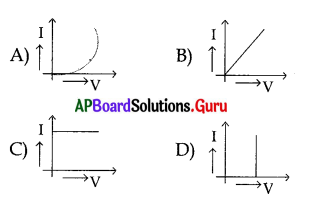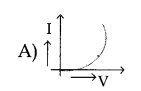Question 93.
Two resistors are connected in ……………….. then same current flows through two resistors.
A) series
B) parallel
C) series and parallel
D) none
A) series

Question 94.
Three resistors 2 Ω, 3 Ω and R Ω are connected in series to a battery of voltage 20 V. If the current through the circuit is 1 ampere then the value of R is ………………… ohm.
A) 5
B) 10
C) 15
D) 25
C) 15

Question 95.
Three resistors each of value 3 Ω are connected, in parallel combination. Their equivalent resistance is …………………….
A) 9 Ω
B) 1 Ω
C) 0.33 Ω
D) 1.5 Ω
B) 1 Ω

Question 96.
At constant temperature the ratio of potential difference to current is not constant for the following …………………….
A) iron
B) copper
C) light emitting diode
D) aluminium
C) light emitting diode

Question 97.
If the potential difference in a circuit is 240 V and the resistance is 60 Ω then the current flowing through the circuit is …………………….
A) 300 A
B) 180 A
C) 0.25 A
D) 4 A
A) 300 A

Question 98.
Three resistors of resistances 3 Ω, 4 Ω and 6 Ω are connected in parallel. The combination is connected to a battery of 8 V. The current in the circuit is ……………………
A) 4 A
B) 3 A
C) 6 A
D) 8 A
C) 6 AQuestion 99.
Which one of the following material has high resistivity
A) silicon
B) wet wood
C) glass
D) rubber
D) rubber

Question 100.
The equivalent resistance is 8 Ω when two resistances are connected in series and 1.5 Ω when they are connected in parallel then the values of these resistances is ……………………….
A) 2, 0.75
B) 6, 0.75
C) 0.75, 0.75
D) 2, 6
D) 2, 6

Question 101.
a) Semi conductors obey the Ohm’s law.
b) Metallic conductors obey the Ohm’s law.
A) only a is true
B) only b is true
C) both a and b are true
D) both a and b are false.
B) only b is true

Question 102.
Which among the following materials have their resistivity of the order of 1014 to 1016 Ω – m
A) conductors
B) semiconductors
C) insulators
D) all
C) insulators

Question 103.
Which one among the following statements is true ?
A) Resistance of a conductor is independent of it’s length
B) Resistance of a conductor is directly proportional to its length
C) Resistance of a conductor is inversely proportional to its length
D) Resistance of a conductor is indepen-dent of its temperature.
B) Resistance of a conductor is directly proportional to its length

Question 104.
Volt per ampere is called
A) watt
B) coloumb
D) ohm
D) ohm

Question 105.
Which among the following quantities has the unit ohm-meter
A) Resistance
B) Resistivity
C) Charge
D) Potential
B) Resistivity

Question 106.
Which among the material has greater resistivity at room temperature ?
A) Iron
B) Glass
C) Gold
D) Germanium
B) GlassQuestion 107.
Three resistors each of 6 Ω are connected in the form of a triangle. The resistance across any two corners of the triangle is
A) 0.25 Ω
B) 6 Ω
C) 4 Ω
D) 18 Ω
C) 4 Ω

Question 108.
A potential difference of 40 V is maintained across a conductor of resistance 20 Ω at constant temperature. The current passing through it is in amperes …………………..
A) 0.05
B) 80
C) 20
D) 2
D) 2

Question 109.
Statement X : R1, R2 and R3 resistors are connected in series. The equivalent resistance R = R1 + R2 + R3.
Statement Y : R1, R2 and R3 resistors are connected in parallel. The reciprocal of equivalent resistance $$\frac{1}{\mathbf{R}}=\frac{1}{\mathbf{R}_{1}}+\frac{1}{\mathbf{R}_{2}}+\frac{1}{\mathbf{R}_{3}}$$
A) Both statements are true
B) Both statements are false
C) X is true, Y is false
D) X is false, Y is true.
A) Both statements are true

CONCEPT – 3 : Kirchhoff’s laws and Electric power

Question 110.
Which of following laws are called Kirchhoff’s laws ?
A) Junction law
B) Loop law
C) Both A and B
D) None
C) Both A and B

Question 111.
Which of the following Kirchhoff’s law is based on the conservation of charge?
A) Junction law
B) Loop law
C) Both A and B
D) None
A) Junction law

Question 112.
Which of the following Kirchhoff’s law is based on the conservation of energy?
A) Junction law
B) Loop law
C) Both A and B
D) None
B) Loop law

Question 113.
Statement X : At any Junction point in a circuit where the current divides the sum of the currents into the junction must equal the sum of the currents leaving the junction.
Statement Y : The algebraic sum of the increases and decreases in potential differences across various components of a closed circuit loop must be zero.
A) Both statements are true
B) Both statements are false
C) X is true, Y is false
D) X is false, Y is true.
A) Both statements are true

Question 114.
Statement X : According to Junction law there is no accumulation of electric charges at any junction point in a circuit.
Statement Y : According to Loop law the algebraic sum of changes in potential differences is equal to zero when we have completely traversed the circuit loop.
A) Both statements are true
B) Both statements are false
C) X is true, Y is false
D) X is false, Y is true.
A) Both statements are trueQuestion 115.
Statement X : emf of a battery is taken as positive when we move from negative terminal to positive terminal across the battery in a closed circuit loop.
Statement Y : emf of a battery is taken as positive when we move from positive terminal to negative terminal across the battery in a closed circuit loop.
A) Both statements are true
B) Both statements are false
C) X is true, Y is false
D) X is false, Y is true.
C) X is true, Y is false

Question 116.
Statement X : The potential difference across the resistor is taken as negative when we move along the direction of electric current in a closed circuit.
Statement Y : The potential difference across the resistor is taken as positive when we move against the direction of electric current in a closed circuit.
A) Both statements are true
B) Both statements are false
C) X is true, Y is false
D) X is false, Y is true.
A) Both statements are true

Question 117.
Apply Kirchhoff’s Junction law and find i3 value of the following figure.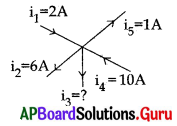A) 19 A
B) 5 A
C) 2 A
D) 1 A
B) 5 A

Question 118.
Read the following statement and indicate which law states that “There is no accumulation of electric charge at any junction in an electric circuit.”
A) Kirchhoff’s Junction law
B) Kirchhoff’s Loop law
C) Ohm’s law
D) Coloumb’s law
A) Kirchhoff’s Junction law

Question 119.
In the figure the potential at A is ……………………. when the potential at B is zero.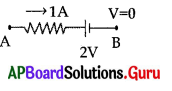A) 5 V
B) 7 V
C) 2 V
D) 0V
B) 7 V

Question 120.
According to Kirchhoff’s laws which of the following equation is correct from the figure ?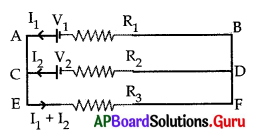A) For the loop ACDBA
-V2 + I2R2 – I1R1 + V1 = 0
B) For the loop EFDCE
-(I1 + I2)R3 – I2R2 + V2 = 0
C) For the loop EFBAE
-(I1 + I2)R3 – I1R1+ V1 = 0
D) All of the above
D) All of the above

Question 121.
Consider the following statements.
X : In series connection the same current flows through each element.
Y : In parallel connection the same potential difference gets applied across each element.
A) Both X and Y are correct
B) X is correct but Y is wrong
C) X is wrong but Y is correct
D) Both X and Y are wrong
A) Both X and Y are correctQuestion 122.
The equation for electric power is …………………….
A) P = VI
B) P = I2R
C) P = V2/R
D) All
D) All

Question 123.
1 Volt × 1 Ampere = ………………….
A) 1 – watt
B) 1 – volt
C) 1 – ampere
D) 1 – ohm
A) 1 – watt

Question 124.
The S.I unit of electric power is ………………………
A) watt
B) kilo watt
C) kilo watt hour
D) watt hour
A) watt

Question 125.
The kilo watt hour is the unit of ……………………..
A) electric power
B) electrical energy
C) electrical current
D) none
B) electrical energy

Question 126.
1 KWH = ……………………
A) 36 × 106 J
B) 3.6 × 105 J
C) 3.6 × 106 J
D) 36 × 10 5J
C) 3.6 × 106 J

Question 127.
The current in the wire depends …………………..
A) only on the potential difference applied
B) only on the resistance of the wire
C) on both of them
D) none of them
C) on both of them
Question 128.
The power delivered by a battery of emf 10 V is 10 W. Then the current delivered
by the battery is …………………
A) 10 A
B) 1 A
C) 100 A
D) 0.1 A
B) 1 AQuestion 129.
Observe the circuit. The power consumed by the resistor R2 is P. The power consumed by the resistor R2 when the resistor R1 is removed from the circuit is …………………. (take R1 = R2)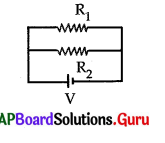A) 2P
B) P/2
C) P
D) 4P
B) P/2

Question 130.
Which of the following is correct regarding electricity
A) P = $$\frac{\mathrm{V}^{2}}{\mathrm{R}}$$
B) P = $$\frac{\mathrm{I}^{2}}{\mathrm{R}}$$
C) P = V2R
D) P = VIR
A) P = $$\frac{\mathrm{V}^{2}}{\mathrm{R}}$$

Question 131.
A bulb is marked 70 W and 210 V. The resistance is ………………….
A) 210 Ω
B) 70 Ω
C) 280 Ω
D) 630 Ω
D) 630 Ω

Question 132.
An electric bulb is marked 40 W and 120 V. It means that in one second it converts …………………….
A) 120 J of electric energy into heat or light
B) 40 J of electric energy into heat or light
C) 160 J of electric energy into heat or light
D) 3 J of electric energy into heat or light
B) 40 J of electric energy into heat or light

Question 133.
A bulb of resistance 200 Ω is connected to a 10 V battery. The power consumption is ……………………
A) 2 W
B) 20 W
C) 0.5 W
D) 0.05 W
C) 0.5 W

Question 134.
Which of the following is used to prevent damages due to overloading to the household circuit ?
A) switch
B) fuse
C) resistance
D) all
B) fuse

Question 135.
The unit generally used for power consumption is …………………..
A) kilo watt
B) volt
C) ampere
D) ohm
A) kilo watt

Question 136.
In an electric circuit fuse is connected in …………………..
A) series to neutral wire
B) series to live wire
C) parallel to neutral wire
D) parallel to live wire
B) series to live wireQuestion 137.
An electric bulb of 360 Ω resistance is connected to a 6 V battery. The power consumption is ………………………
A) 0.1 W
B) 3 W
C) 3W
D) 20 W
A) 0.1 W

Question 138.
If V is the voltage, R is the resistance and I is the current then the equation for electric power is …………………….
A) $$\frac{\mathrm{V}^{2}}{\mathrm{R}}$$
B) IR2
C) V2R
D) $$\frac{\mathrm{V}}{\mathrm{I}}$$
A) $$\frac{\mathrm{V}^{2}}{\mathrm{R}}$$

Question 139.
A) Increasing the number of electric appliances consuming power more than 4.8 KW
B) The household circuits draw the current from mains more than 20 A
C) The household circuits draw the current from mains more than 40 A
D) Both A and B
D) Both A and B

Question 140.
In an experiment verification of Ohm’s law following observations are obtained.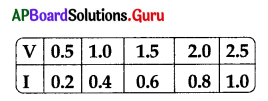Choose the correct statement from the following.
A) V = 1.25 V when I = 0.5A
B) I = 0.3A when V = 0.75 A
C) Resistance in circuit is 2.5 £2
D) All
D) All

Question 141.
Two bulbs have ratings 100 W, 220 V and 60 W, 220 V. Which of the following statement is true.
A) Two bulbs have same resistance
B) The bulb 100 W has greater resistance than 60 W
C) The bulb 60 W has greater resistance than 100 W.
D) None
C) The bulb 60 W has greater resistance than 100 W.

Question 142.
A house has 3 tube lights and two fans. Each tube light draws 40 W and each fan draws 80 W. On the average all the tube lights and fan work 5 hours every day. Find the electrical energy consumed in 30 days.
A) 1 KWH
B) 2 KWH
C) 52 KWH
D) 52000 KWH